# Chapter 8 Chemical Equations ReactionsRxns Chemical ReactionsRxns Chemical

• Slides: 23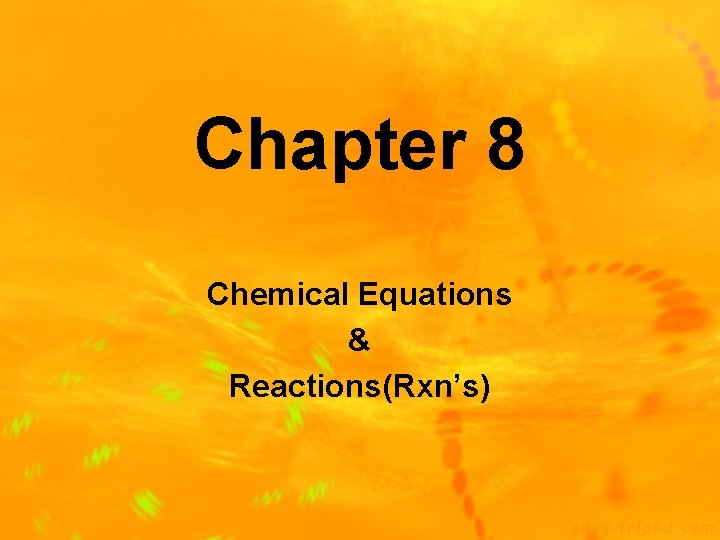Chapter 8 Chemical Equations & Reactions(Rxn’s)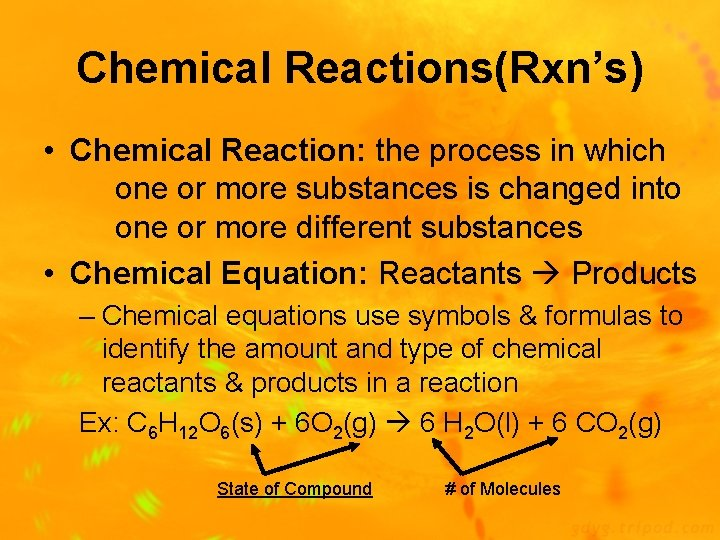Chemical Reactions(Rxn’s) • Chemical Reaction: the process in which one or more substances is changed into one or more different substances • Chemical Equation: Reactants Products – Chemical equations use symbols & formulas to identify the amount and type of chemical reactants & products in a reaction Ex: C 6 H 12 O 6(s) + 6 O 2(g) 6 H 2 O(l) + 6 CO 2(g) State of Compound # of MoleculesCharacteristics of Chemical Reactions • 1) A chemical equation must represent all reactants and products • 2) A chemical equation must contain correct formulas for reactants and products • 3) Conservation of mass must be expressed in the equation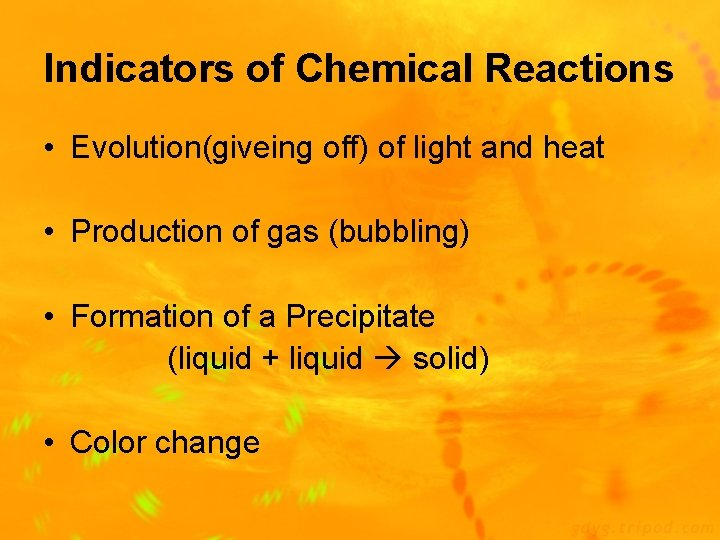Indicators of Chemical Reactions • Evolution(giveing off) of light and heat • Production of gas (bubbling) • Formation of a Precipitate (liquid + liquid solid) • Color change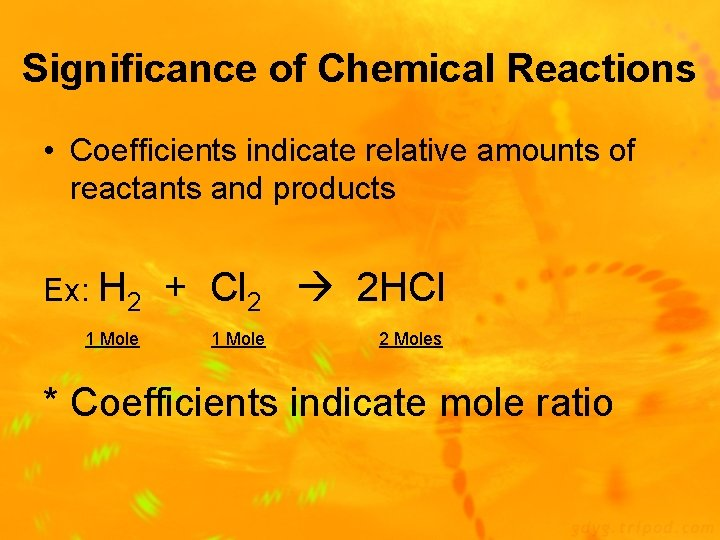Significance of Chemical Reactions • Coefficients indicate relative amounts of reactants and products Ex: H 2 1 Mole + Cl 2 2 HCl 1 Mole 2 Moles * Coefficients indicate mole ratioSymbols Used in Chemical Reactions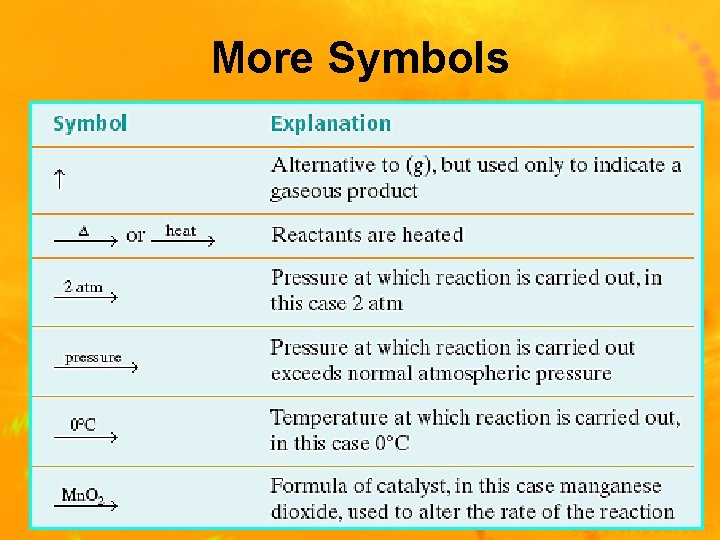More Symbols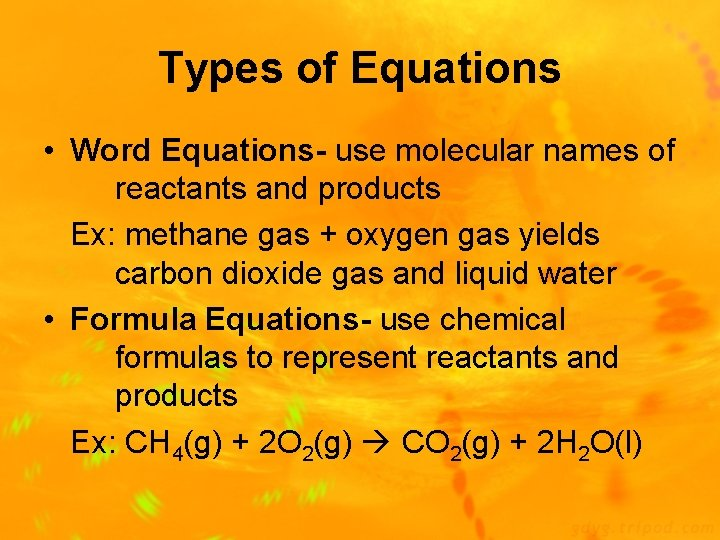Types of Equations • Word Equations- use molecular names of reactants and products Ex: methane gas + oxygen gas yields carbon dioxide gas and liquid water • Formula Equations- use chemical formulas to represent reactants and products Ex: CH 4(g) + 2 O 2(g) CO 2(g) + 2 H 2 O(l)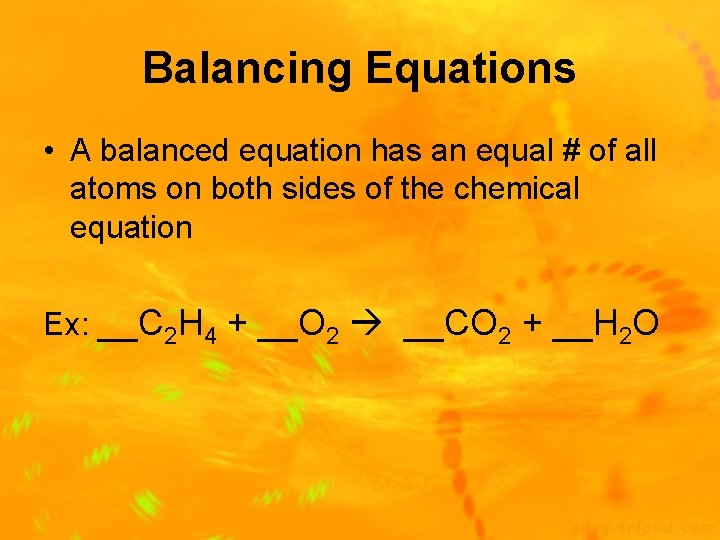Balancing Equations • A balanced equation has an equal # of all atoms on both sides of the chemical equation Ex: __C 2 H 4 + __O 2 __CO 2 + __H 2 OTypes of Chemical Reactions 1) Synthesis Reaction: 2 or more substances combine to form 1 new compound (A + X AX) Ex: 2 Mg(s) + O 2(g) 2 Mg. O(s) 2) Decomposition Reaction: 1 substance breaks down into 2 new substances (AX A + X) Ex: Ca. CO 3(s) Ca. O(s) + CO 2(g)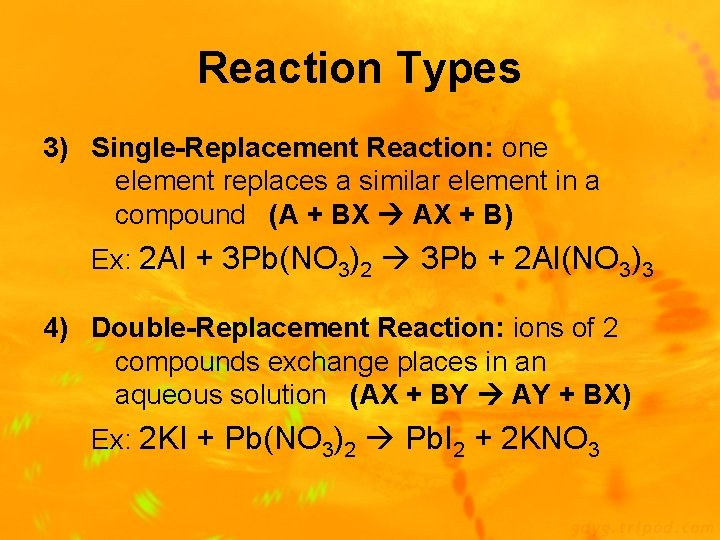Reaction Types 3) Single-Replacement Reaction: one element replaces a similar element in a compound (A + BX AX + B) Ex: 2 Al + 3 Pb(NO 3)2 3 Pb + 2 Al(NO 3)3 4) Double-Replacement Reaction: ions of 2 compounds exchange places in an aqueous solution (AX + BY AY + BX) Ex: 2 KI + Pb(NO 3)2 Pb. I 2 + 2 KNO 3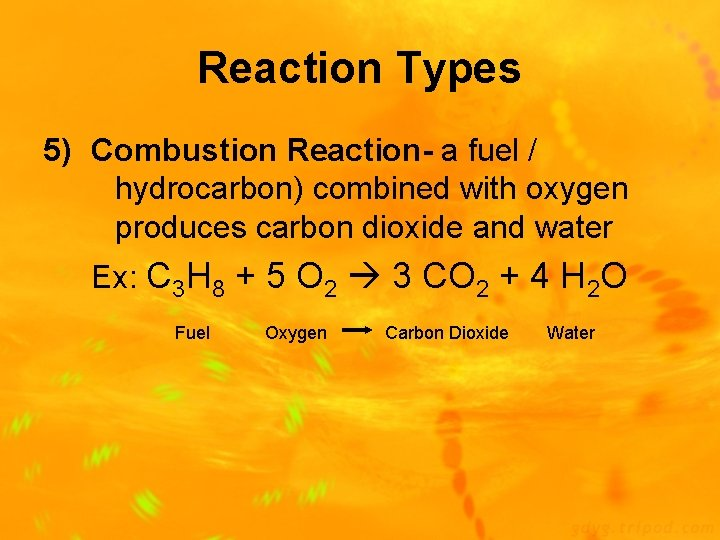Reaction Types 5) Combustion Reaction- a fuel / hydrocarbon) combined with oxygen produces carbon dioxide and water Ex: C 3 H 8 + 5 O 2 3 CO 2 + 4 H 2 O Fuel Oxygen Carbon Dioxide WaterDetermining Reaction Types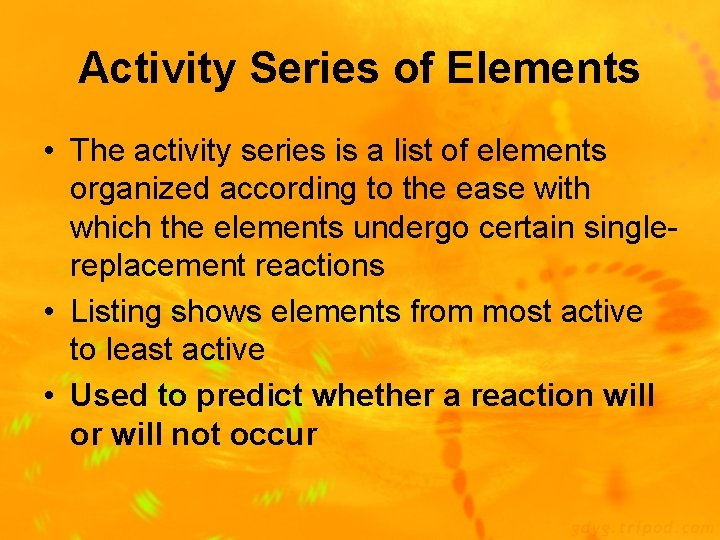Activity Series of Elements • The activity series is a list of elements organized according to the ease with which the elements undergo certain singlereplacement reactions • Listing shows elements from most active to least active • Used to predict whether a reaction will or will not occur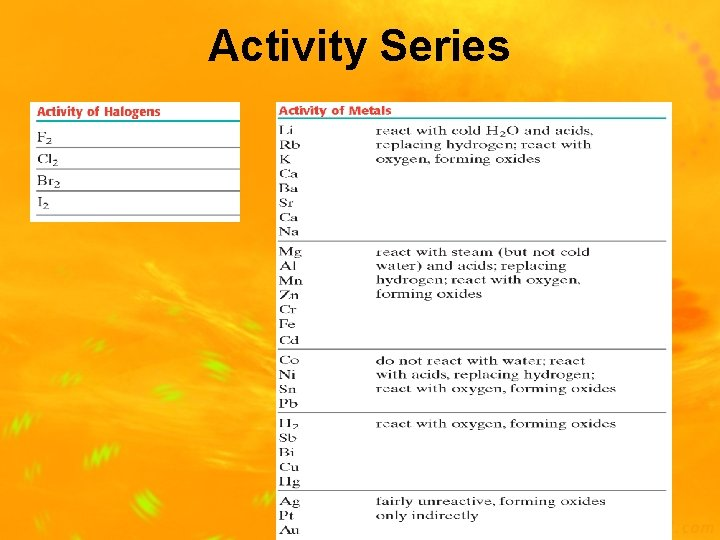Activity Series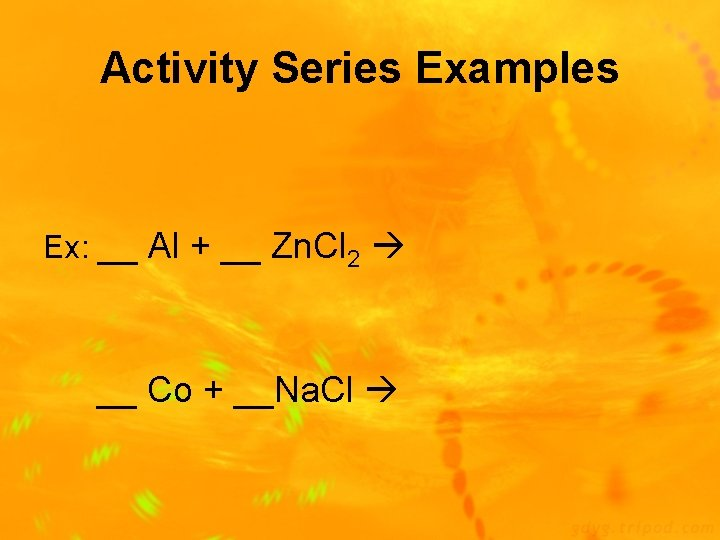Activity Series Examples Ex: __ Al + __ Zn. Cl 2 __ Co + __Na. Cl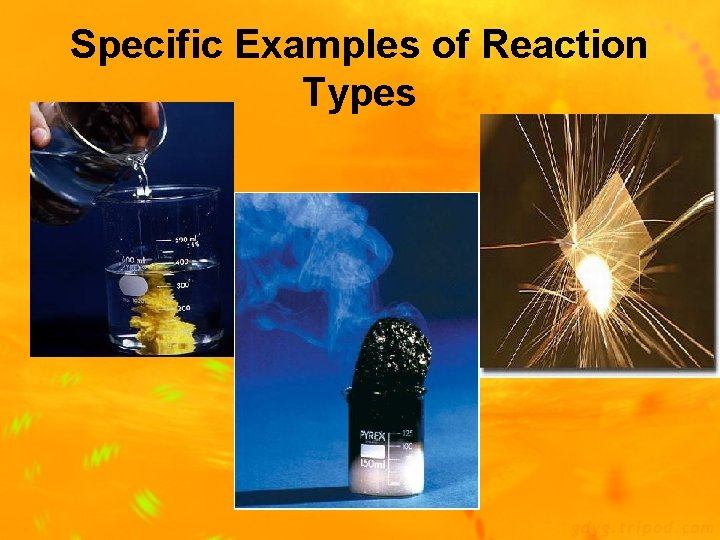Specific Examples of Reaction Types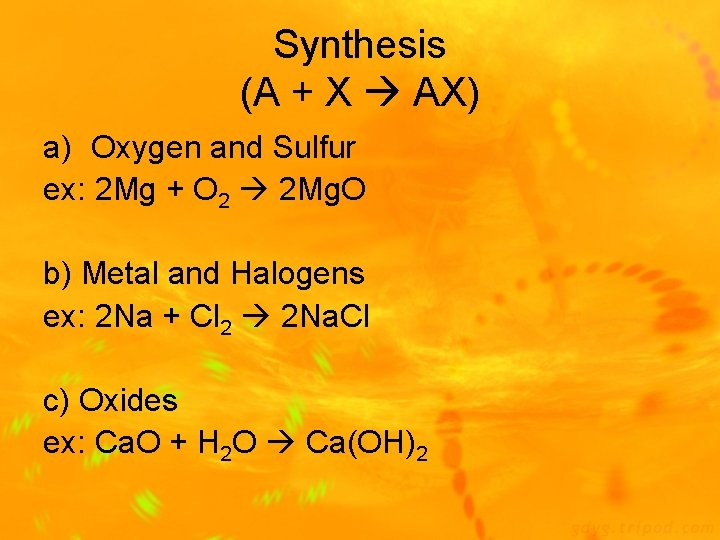Synthesis (A + X AX) a) Oxygen and Sulfur ex: 2 Mg + O 2 2 Mg. O b) Metal and Halogens ex: 2 Na + Cl 2 2 Na. Cl c) Oxides ex: Ca. O + H 2 O Ca(OH)2Decomposition (AX A + X) a) Binary Compounds ex: 2 Hg. O 2 Hg + O 2 b) Metal Carbonates ex: Ca. CO 3 Ca. O + CO 2 c) Metal Hydroxides ex: Ca(OH)2 Ca. O + H 2 O d) Metal Chlorates ex: 2 KCl. O 3 2 KCl + 3 O 2 e) Acids ex: H 2 SO 4 SO 3 + H 2 OSingle Replacement (A + BX AX + B) a) Metal replaced by metal ex: 2 Al + 3 Pb(NO 3)2 3 Pb + 2 Al(NO 3)3 b) Hydrogen replaced by metal ex: 3 Fe + 4 H 2 O Fe 3 O 4 + 4 H 2 c) Acid Hydrogen replaced by metal ex: Mg + 2 HCl H 2 + Mg. Cl 2 d) Replacement of halogens ex: Cl 2 + 2 KBr 2 KCl + Br 2Double Replacement (AX + BY BX +AY) a) Formation of a precipitate ex: 2 KI + Pb(NO 3)2 Pb. I 2 + 2 KNO 3 b) Formation of gas ex: Fe. S + 2 HCl H 2 S + Fe. Cl 2 c) Formation of water ex: HCl + Na. OH Na. Cl + H 2 O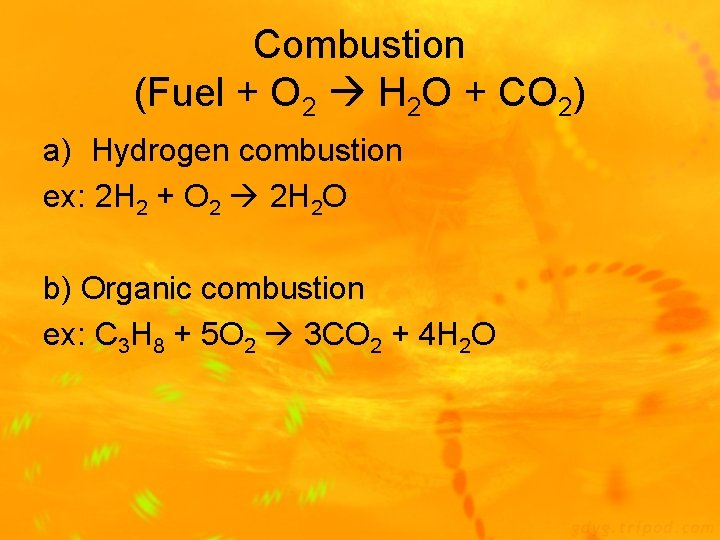Combustion (Fuel + O 2 H 2 O + CO 2) a) Hydrogen combustion ex: 2 H 2 + O 2 2 H 2 O b) Organic combustion ex: C 3 H 8 + 5 O 2 3 CO 2 + 4 H 2 OEND OF CHAPTER 8 NOTES!!!